﻿ Robust Goodness of Fit Test Based on the Forward Search

### Robust Goodness of Fit Test Based on the Forward Search

Abbas MahdaviOPEN ACCESSPEER-REVIEWED

## Robust Goodness of Fit Test Based on the Forward Search

Department of Statistics, Faculty of Mathematical Sciences, Vali-e-Asr University of Rafsanjan, Rafsanjan, Iran

### Abstract

The most frequency used goodness of fit tests are based on measuring the distance between the theoretical distribution function and the empirical distribution function (EDF), but presence of outliers influences these tests strongly. In this study, we propose a simple robust method for goodness of fit test by using the Forward Search (FS) method. The FS method is a powerful general method for identifying outliers and their effects on the hypothesized model. The performance and the ability of the procedure to capture the structure of data, even in the presence of outliers, are illustrated by some simulation studies and real data examples.

### At a glance: Figures

12
Prev Next

• Mahdavi, Abbas. "Robust Goodness of Fit Test Based on the Forward Search." American Journal of Applied Mathematics and Statistics 1.1 (2013): 6-10.
• Mahdavi, A. (2013). Robust Goodness of Fit Test Based on the Forward Search. American Journal of Applied Mathematics and Statistics, 1(1), 6-10.
• Mahdavi, Abbas. "Robust Goodness of Fit Test Based on the Forward Search." American Journal of Applied Mathematics and Statistics 1, no. 1 (2013): 6-10.

 Import into BibTeX Import into EndNote Import into RefMan Import into RefWorks

### 1. Introduction

Model testing and verification are important part of statistical inference to obtain information about the population from which the sample is drawn. Most of the parametric tests require a number of assumptions such as normality. These assumptions should be validated before we go advance with other aspects of statistical inference. Goodness of fit test measures the degree of agreement between the distribution of an observed sample data and a hypothetical statistical distribution. In practice, it often happens that an assumption such normality holds approximately in majority of observations, but some observations follow a different pattern or no pattern at all. Such atypical data are called outliers. A single outlier can even have a large disturbing effect on a classical statistical method that is optimal under the classical assumptions.

The Forward Search (FS) approach is a powerful general method that provides diagnostic plots for finding outliers and determining their effect on the fitted models. The FS method starts from a small, robustly chosen subset of the data and increase the subset size until finally all the data are fitted. The outliers enter the model in the last steps and the entrance point of the outliers can be exposed by monitoring some statistics of interest during the process. Initially Hadi  and Atkinson  presented the method of fitting a model to subsets of an increasing size for multivariate data analysis. Hadi and Simonoff  used the FS in regression, and the development of the FS was introduced by Atkinson and Riani [4, 5, 6] and Atkinson et al.  for regression and multivariate procedure with a recent discussion in . Recently the FS method is implemented in wide applications, e.g. ANOVA framework , testing normality , finding an unknown number of multivariate outliers , detecting atypical observations in financial data , robust estimation of efficient mean–variance frontiers  and benchmark testing of algorithms for very robust regression .

The purpose of this article is to adopt the FS method in the goodness of fit test for continues variable. The most frequently used goodness of fit tests are based on measuring the distance between the theoretical distribution function and the empirical distribution function (EDF) such as Kolmogorov-Smirnov , Cramer , Anderson-Darling , but presence of outliers influences these tests strongly. In this paper we try to determine how many and which observations agree with the null hypothesis distribution. In order to adapt the FS for goodness of fit test, we need a way to select an outlier free subset and a test for goodness of fit to be used in the search.

The paper is organized as follows. Section 2 presents the proposed forward search algorithm in goodness of fit test. In Section 3, the performance of the method is illustrated with simulated data and the behavior of our procedure is analyzed. Finally in Section 4 we show an application of the proposed method to real world data by using of the blood clotting data set. Concluding remarks are provided in Section 5.

### 2. Forward Search in Goodness of Fit Test

Many goodness of tests for testing hypotheses about specified distribution are available in literature, some of them are special purpose tests, and they are appropriate and perform well only for some special situations. Anderson-Darling test gives more attention to the tails of distribution, hence it can be useful for investigated the effects of outliers on the goodness of fit test, since the outliers have low probability when they originate from the same statistical distribution as the other observations. For further results about Anderson-Darling test see .

In this paper we use FS method not only to detecting outliers, but also for investigating the effect of outliers on the Anderson-Darling goodness of fit test. The FS method has three steps: the first step is choosing outlier free subset of all observations, the second step presents the plan to progressing in FS and the last step is monitoring statistics during the search. In the following subsections we adopt these three points separately.

2.1. Step 1: Choice of the Initial Subset

Starting point of the FS procedure is choosing outlier free subset of observations robustly. If the vector of ordered observationscomes from a distribution functionwith known parameters, then it can be shown that the probability distribution functions (pdf) of the random variables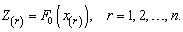(1)

are given by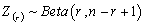, so its mean is(2)

It is possible to use of transformations of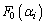to estimate the value of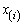. It means that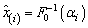, where the evaluation of quantile function may be involves numerical methods and it is done for common distributions in many statistical package. Therefore we obtain the vector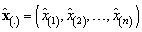of estimated expected values of the ordered sample. Now the elements of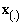are reordered according their absolute residuals from estimated values, denoted by.

First the size of initial subset must be specified. A larger initial subset will give more stable estimates and smoother forward plots. Also, it is irrational that more than half of the data be outliers, hence we set the size of initial subset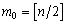. The initial subset can be achieve by choosing the firstobservations of the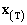that are closer to the their estimated values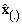.

2.2. Step 2: Adding Observations during the FS

After choosing initial subset, in the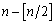remaining steps all observations must be add to it. At each step, the observations that are closer to the previously fitted model are added to the subset. The parameters of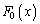are completely known and we try to find the largest subset of observations that can be distributed from, it is not necessary to reorder the observationsat each step of the search. Therefore we add the next observation ofto the previously chosen subset in each step of the search.

2.3. Step 3: Monitoring the Search

For detecting and determining the effect of outliers, some interested statistics of interest must be monitor during the search. Let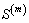be the subset of the first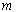observations of. The collections of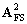statistics of the subsetduring the FS procedure defined as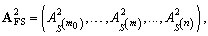(3)

where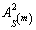denote the value of Anderson-Darling test statistic for the subsetin testing the null hypothesisagainst the alternative hypothesis, whereis completely known and defines hypothesized distribution function to be test. To obtain the corresponding rejection region of the (3) during the search, the empirical quantiles of this statistic must be estimated by simulation study in each step of the search and for different sample sizes.

### 3. Simulation Study

For evaluate the proposed statistic (3), we conduct simulation studies that aim to consider the behavior of this statistic in the presence of outliers and ability of FS for detecting them. Eight samples are considered which are generated in the following way:

• Sample A: 100 observations are generated from a standard normal distribution.

• Sample B: 95 observations are generated from a standard normal distribution and for contamination 5 observations are generated from a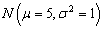.

• Sample C: 100 observations are generated from an.

• Sample D: 95 observations are generated from an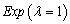and for contamination 5 observations are generated from a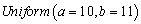.

• Sample E: 100 observations are generated from a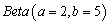.

• Sample F: 95 observations are generated from aand for contamination 5 observations are generated from a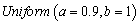.

• Sample G: 100 observations are generated from a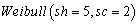.

• Sample H: 95 observations are generated from aand for contamination 5 observations are generated from a.

For each sample, we are interested in testing the null hypothesis that majority of observations comes from it. In Figure 1, values ofduring the search are plotted for samples A-H and compared with corresponding 95% quantile of its distribution obtained from a simulation study with clean data. The null hypothesis is accepted in each step of the search for clean samples A, C, E and G, and it’s rejected after entrance of outliers in the last steps for contaminated samples B, D, F and H.

Figure 1. Forward plots ofduring the search for samples A-H
3.1 Empirical Power ofIn this subsection, we interest to evaluate the empirical power of our approach. Two following examples are considered:

Example 1: Consider the null hypothesisagainst. Figure 2 shows the empirical power of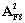against following alternative hypotheses by generating 10000 samples of size 100.

(a)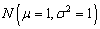(b)(c)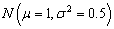(d)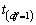Figure 2. Empirical power offor example 1 versus alternative distributions (a-d)

Example 2: Here, we analysed the estimated power of testing the null hypothesisagainst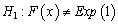, by regarding the following alternative distributions, the results are shown in Figure 3.

(e)(f)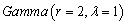(g)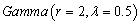(h)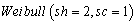Figure 3. Empirical power offor example 2 versus alternative distributions (e-h)

After analysis the two mentioned examples, we concluded that the power of our proposed procedure in first steps of the search is low for close alternatives. It is due to our aim is to find the largest subset of observations that can be distributed as the null hypothesis and for close alternatives this can occurs in the first steps of the search. Therefore, the larger sample size provides safer procedure to detect and investigate the effect of outliers.

### 4. The Blood Clotting Data

To investigate the performance of the proposed approach to real-world data, we use the blood clotting dataset relating to blood clotting activity (PCA) is measured for 158 Norway rats before (baseline) and four days after injection of an anticoagulant, published by Heiberg . This data set contains 91 instances for female gender, for our purposes, we use the blood clotting activity at baseline (PCA0) for the female gender.

The histogram and boxplot for PCA0, plotted in Figure 4, show some observations that could be regard as outliers. To test normality assumption for these observations, we use the robust M-estimator  to estimate parameters, that are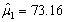and. Therefore, we perform Test 1 as: the null hypothesis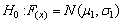against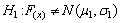and the results of forward search plotted in Figure 5 (a).

Figure 5. (a) Forward search results for Test 1 and (b) Forward search results for Test 2

The null hypothesis of Test 1 accepted in each step except the last steps due to entrance of outliers after steps 85 onwards, indicating that 6 observations are important outliers. After removing these outliers, the maximum likelihood estimate of parameters areand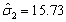. Now we have the estimated parameters that are more optimal respect to M-estimator with total observations and hence we define Test 2 to verify the distribution of the remained observations as follow: the null hypothesisagainst. Figure 5 (b) shows the ability of our proposed approach to detecting outliers and finding the largest subset of observations that verify the null hypothesis.

### 5. Concluding Remarks

In this paper, a new robust approach to the goodness of fit test for continuous distributions has been presented. Our work concerns the effect of outliers in goodness of fit and the robust FS method is implemented to individuate the outliers. At every step of the FS, the proposed robust statistics are computed and this search method divides the group of outliers from the other observations by a graphical approach. Hence, it is able to determine whether the majority of data is distributed as the null hypothesis distribution. In order to illustrate the application and the advantage of the FS approach we conducted simulation studies. Furthermore, we showed an application of the proposed approach to real data.

### References

  Hadi, A. S., Identifying multiple outliers in multivariate data, Journal of the Royal Statistical Society, Series B, 54. 761-771. 1992.In article  Atkinson, A. C., Fast very robust methods for the detection of multiple outliers, Journal of the American Statistical Association, 89. 1329-1339. 1994.In article  Hadi, A. S., Simonoff, J. S., Procedures for the identification of multiple outliers in linear models, Journal of the American Statistical Association, 88. 1264-1272. 1993.In article  Atkinson, A. C., Riani, M., Robust Diagnostic Regression Analysis, Springer, New York, 2000.In article  Atkinson, A. C., Riani, M., Forward search added-variable t-tests and the effect of masked outliers on model selection, Biometrika, 89(4). 939-946. 2002.In article  Atkinson, A. C., Riani, M., The Forward search and data visualization, Computational Statistics, 19. 29-54. 2004.In article  Atkinson, A. C., Riani, M., Cerioli, A., Exploring Multivariate Data with the Forward Search, Springer, New York, 2004.In article  Atkinson, A. C., Riani, M., Cerioli, A., The forward search: theory and data analysis, Journal of the Korean Statistical Society, 39. 117-134. 2010.In article  Bertaccini, B., Varriale, R., Robust Analysis of Variance: an approach based on the Forward, Computational statistics and data analysis, 51. 5172-5183. 2007.In article  Coin, D., Testing normality in the presence of outliers, Statistical Methods & Applications, 17. 3-12. 2008.In article  Riani, M., Atkinson, A.C., Cerioli, A., Finding an unknown number of multivariate outliers, Journal of the Royal Statistical Society Series, B 71. 447-466. 2009.In article  Bellini, T., Detecting atypical observations in financial data: the forward search for elliptical copulas, Advances in Data Analysis and Classification, 4. 287-299. 2010.In article  Grossi, L., Laurini, F., Robust estimation of efficient mean-variance frontiers, Advances in Data Analysis and Classification, 5. 3-22. 2011.In article  Torti, F., Perrotta, D., Atkinson, A.C., Riani, M., Benchmark testing of algorithms for very robust regression: FS, LMS and LTS, Computational statistics and data analysis, 56. 2501-2512. 2012.In article  Kolmogorov, A. N., Sulla Determinazione Empirica di Una Legge di Distribuzione, Giornale dell’Istituto Italiano degli Attuari, 4. 83-91. 1933.In article  Cramér, H., On the composition of elementary errors, Scandinavian Actuarial Journal, 11. 141-180. 1928.In article  Anderson, T. W., Darling, D. A., A Test of Goodness of Fit, Journal of the American Statistical Association, 49. 765-769. 1954.In article  Heiberg, A-C., Project at The Royal Veterinary and Agricultural University, 1999.In article  Huber, P.J., Robust Statistics, John Wiley & Sons, New York, 1981.In article